# JP2006308649A - Imaging apparatus - Google Patents

## Info

Publication number
JP2006308649A
JP2006308649A JP2005127787A JP2005127787A JP2006308649A JP 2006308649 A JP2006308649 A JP 2006308649A JP 2005127787 A JP2005127787 A JP 2005127787A JP 2005127787 A JP2005127787 A JP 2005127787A JP 2006308649 A JP2006308649 A JP 2006308649A
Authority
JP
Japan
Prior art keywords
lens group
lens
lt
group
positive
Prior art date
Legal status (The legal status is an assumption and is not a legal conclusion. Google has not performed a legal analysis and makes no representation as to the accuracy of the status listed.)
Pending
Application number
JP2005127787A
Other languages
Japanese (ja)
Other versions
JP2006308649A5 (en
Inventor
Tsunaki Hozumi

Original Assignee
Olympus Imaging Corp
オリンパスイメージング株式会社
Priority date (The priority date is an assumption and is not a legal conclusion. Google has not performed a legal analysis and makes no representation as to the accuracy of the date listed.)
Filing date
Publication date
Application filed by Olympus Imaging Corp, オリンパスイメージング株式会社 filed Critical Olympus Imaging Corp
Priority to JP2005127787A priority Critical patent/JP2006308649A/en
Priority claimed from US11/404,781 external-priority patent/US7333274B2/en
Publication of JP2006308649A publication Critical patent/JP2006308649A/en
Publication of JP2006308649A5 publication Critical patent/JP2006308649A5/ja
Application status is Pending legal-status Critical

• 238000003384 imaging method Methods 0 abstract title 4
• 230000003405 preventing Effects 0 abstract 1

## Images

•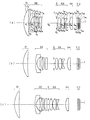•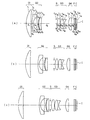•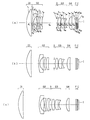•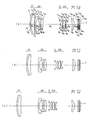•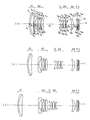•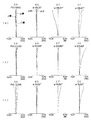•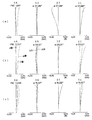•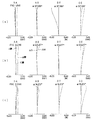•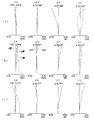•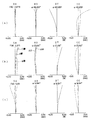•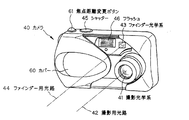••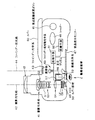## Abstract

PROBLEM TO BE SOLVED: To provide an image pickup apparatus provided with a zoom lens that appropriately prevents the enlargement of a lens system due to a wide angle by appropriately configuring the power arrangement of each group and obtains good imaging performance even with a large aperture.
A positive first group G1, a negative second group G2, a positive third group G3, and a positive fourth group G4, and at the time of zooming from the wide angle end to the telephoto end, At least the first group, the second group, the third group, so that there is an increase between the second group, a decrease between the second group and the third group, and an increase between the third group and the fourth group. In the image pickup apparatus including the zoom lens in which the group moves, the third group G3 includes a positive lens, a positive lens, and a negative lens.
[Selection] Figure 1

## Description

The present invention relates to an image pickup apparatus, and more particularly to an image pickup apparatus including a wide-angle zoom lens that is small but advantageous for achieving a high zoom ratio.

In a compact digital camera optical system, a compact negative leading type is known, but it is difficult to increase the zoom ratio or increase the aperture. Therefore, the positive leading type is used in an optical system that is required to have a higher zoom ratio and a larger aperture (Patent Document 1, Patent Document 2, and Patent Document 3).
JP 2004-212618 A JP 2004-252204 A JP-A-9-61714

However, in the conventional forward type, when trying to obtain a wide angle of view, the diameter of the front lens becomes large, and miniaturization and cost reduction have not been sufficiently achieved.

The present invention has been made in view of such a situation in the prior art, and the object thereof is to prevent the enlargement of the lens system due to widening of the angle by appropriately configuring the power arrangement of each group, and to increase the aperture. However, an object of the present invention is to provide an imaging apparatus including a zoom lens that obtains good imaging performance.

The image pickup apparatus of the present invention that achieves the above object includes, in order from the object side, a positive first lens group, a negative second lens group, a positive third lens group, and a positive fourth lens group, from the wide-angle end. At the time of zooming to the telephoto end, the space between the first lens group and the second lens group increases, the space between the second lens group and the third lens group decreases, and the third lens group and At least the first lens group, the second lens group, and the third lens group are moved so that the space between the fourth lens group and the fourth lens group increases, and the first lens group includes a single lens or a cemented lens, In an imaging apparatus including a zoom lens that is positioned on the image side at the wide-angle end than the telephoto end and satisfies the following conditional expressions (A) and (B):
The third lens group includes a positive lens, a positive lens, and a negative lens in order from the object side.

-0.5 <d2 / r1 <2 (A)
0.725 <IH / f r <0.8 ··· (B)
However, d2 is the second lens group and the distance between the third lens group when the one of the focal length f r satisfying conditional expression (B), r1 is a radius of curvature of the most object side surface of the first lens group is there.

Hereinafter, the reason and action of the above configuration in the present invention will be described.

The zoom lens according to the present invention is a four-group zoom lens having positive / negative / positive / positive power arrangement. The negative leading type is disadvantageous for a large aperture and a high zoom ratio. Therefore, such a configuration is adopted in order to reduce the size, increase the aperture, and ensure a zoom ratio.

At the time of zooming from the wide-angle end to the telephoto end, the distance between the first lens group and the second lens group increases, the distance between the second lens group and the third lens group decreases, and the third lens group At least the first lens group, the second lens group, and the third lens group move so that the space between the fourth lens group and the fourth lens group increases.

With this configuration, the front lens diameter is prevented from increasing by having the first lens unit on the image side at the wide-angle end, and the variable magnification burden is shared by both the second lens unit and the third lens unit. Can be doubled and the overall length is shortened.

The first lens group is composed of a single lens or a cemented lens. Since the first lens group has a high beam height of the peripheral luminous flux and has an angle, the front lens diameter is likely to be large, and the aperture is greatly increased due to the increase in the number of lenses.

If the upper limit of 2 of the conditional expression (A) is exceeded, the space between the second lens group and the third lens group becomes large, or the outer diameter of the first lens group becomes large, and the lens system becomes large. If the lower limit of −0.5 is exceeded, a high zoom ratio cannot be secured.

In the present invention, the configuration of the third lens group includes a positive lens, a positive lens, a negative lens, or a positive lens and a cemented lens. By configuring in this way and bringing the principal point position of the third lens group to the incident side,
-To secure a space for zooming on the telephoto side (the space between the second lens group and the third lens group is widened),
-The height of ambient light at the wide-angle end can be kept low, contributing to the miniaturization of the first lens group and the second lens group.

In addition, the third lens group is a lens group that plays an important role not only in zooming but also in image formation. Therefore, the positive, positive, and negative configurations can achieve both aberration correction and downsizing.

With the configuration described above, it is possible to configure a zoom lens having an angle of view of 72 ° or more at the wide-angle end.

In addition to the above configuration, it is desirable that the aperture stop be disposed on the incident side of the third lens group so as to move integrally during zooming.

By disposing the third lens group that is important for the imaging function in the vicinity of the stop, it is possible to reduce the size of the third lens group in the radial direction while providing power. Also, with this configuration, there is little change in the beam height due to zooming, so that stable imaging performance can be obtained over the entire zoom range.

Further, the second lens group may be constituted by three negative meniscus lenses, negative lenses, and positive lenses, or four negative meniscus lenses, negative meniscus lenses, negative lenses, and positive lenses.

By configuring in this way, the space for zooming on the telephoto side is secured by bringing the principal point position of the second lens group to the exit side, and the lateral magnification in the second lens group is increased. It can be set and the zooming effect by the second lens group is enhanced. Moreover, since the entrance pupil position moves to the object side, an increase in the front lens diameter can be prevented.

In the above imaging apparatus, it is preferable that the zoom lens satisfies the following conditional expression (1).

2 <f 4 / f w <3.5 (1)
Here, f 4 is the focal length of the fourth lens group, and fw is the focal length of the entire system at the wide angle end.

Conditional expression (1) is a conditional expression that prescribes an appropriate power of the fourth lens group. When the upper limit of 3.5 is exceeded, the power of the fourth lens group becomes weak and the exit pupil becomes far away. Effect (decreasing the incident angle to the CCD of the image sensor) is weakened, and shading characteristics are deteriorated. In addition, the total length of the lens diameter is increased. When the fourth lens group is used as the focus group, the sensitivity for focus adjustment is reduced, and it is difficult to secure a space. Further, the effect of correcting the spherical aberration fluctuation due to zooming cannot be obtained.

If the lower limit of Conditional Expression (1) is smaller than 2, the back focus is shortened, and a space necessary for inserting the optical filter cannot be secured. Further, the variation of the exit pupil due to zooming becomes large, and the variation of the incident angle to the CCD of the image pickup device becomes large, so it becomes difficult to ensure the shading characteristics over the entire zoom range. In addition, the height of light beams around the first lens group and the second lens group at the wide-angle end increases, leading to an increase in the lens diameter.

It is desirable that the zoom lens satisfies the following conditional expression (2).

0.9 <f 1 / L w <1.2 (2)
Here, f 1 is the focal length of the first lens group, and L w is the total length of the entire system at the wide angle end.

Conditional expression (2) is a conditional expression that defines the relationship between the focal length of the first lens unit and the entire wide-angle end. This conditional expression means that the image formed by the first lens group at the wide-angle end is substantially the same as the position of the image plane by the entire system. As a result, a region with better zooming efficiency can be used in the entire zoom range due to the image formation of the second lens group, so that the space required for zooming can be shortened and the overall length can be reduced.

When the upper limit of 1.2 of the conditional expression (2) is exceeded, the power of the first lens group becomes weak, the imaging position of the light beam incident on the second lens group becomes far, and the lateral magnification in the second lens group Will become smaller. For this reason, the zooming efficiency in the second lens group is deteriorated, the amount of movement of the first lens group during zooming is increased, and the lens unit is increased in size.

If the lower limit of 0.9 in conditional expression (2) is not reached, the power is too strong for the diameter of the first lens group, so that a desired power cannot be obtained with only a single lens or a cemented lens. In addition, since the angle of the peripheral ray at the wide-angle end is large, an increase in the number of lenses causes further enlargement of the optical system.

It is desirable that the zoom lens satisfies the following conditional expression (3).

2.7 <D2 (w) / D3 (w) <5.0 (3)
However, D 2 (w) is the group interval between the second lens group and the third lens group at the wide-angle end, and D 3 (w) is the group interval between the third lens group and the fourth lens group at the wide-angle end.

Conditional expression (3) is an expression that defines an appropriate group spacing arrangement at the wide-angle end. If the upper limit of 5.0 of the conditional expression (3) is exceeded, the height of the light beam incident on and exiting from the first lens group and the second lens group becomes higher, and the front lens becomes larger. Leads to an increase in size. Beyond the lower limit of 2.7, the space for zooming decreases, making it difficult to earn a zoom ratio.

It is desirable that the zoom lens satisfies the following conditional expression (4).

5 <| f 1 / f 2 | <5.7 (4)
Here, f 1 is the focal length of the first lens group, and f 2 is the focal length of the second lens group.

Conditional expression (4) is a conditional expression for appropriately giving a zooming action from the wide-angle end to the telephoto end of the second lens group. If the upper limit of 5.7 in the conditional expression (4) is exceeded, the image side focal position of the first lens group and the object side focal position of the second lens group are separated from each other. Will change the magnification. Therefore, the efficiency at the time of zooming is reduced, the amount of movement due to zooming of the first lens group is increased, and the total length of the telephoto end is increased. Alternatively, the power of the second lens group becomes too strong, and the aberration generated in the second lens group increases. If the lower limit of 5 of the conditional expression (4) is exceeded, the power of the first lens group becomes strong, and it becomes difficult to form a single lens or a cemented lens. In addition, the power of the second lens group is weak and the zooming action is weakened. As a result, the zooming burden for zooming is increased in the third lens group, which leads to an increase in the F number at the telephoto end.

It is desirable that the zoom lens satisfies the following conditional expression (5).

0.6 <f 3 / f 4 <1.2 (5)
Here, f 3 is the focal length of the third lens group, and f 4 is the focal length of the fourth lens group.

Conditional expression (5) is a conditional expression that prescribes the sharing ratio of the imaging action. If the upper limit of 1.2 in conditional expression (5) is exceeded, the imaging action of the third lens group will be weakened and the overall length will be long. Or, since the power of the fourth lens group becomes strong, the variation of the exit pupil position due to zooming becomes large, and it becomes difficult to ensure the shading characteristics. If the lower limit of 0.6 of conditional expression (5) is exceeded, the power of the third lens group will become strong, and the curvature of each lens will become tight, and aberrations cannot be corrected well for large diameters. Alternatively, since the power of the fourth lens group is weakened, the amount of movement at the time of focusing becomes large, and a space cannot be secured.

It is desirable that the zoom lens satisfies the following conditional expression (6).

0.3 <D3 (t) / ft <0.7 (6)
However, D 3 (t) is the group interval, f t of the third lens group and the fourth lens group at the telephoto end is the focal length of the entire system at the telephoto end.

Conditional expression (6) is a conditional expression that defines the power arrangement at the telephoto end. If the upper limit of 0.7 in conditional expression (6) is exceeded, the height of the light beam on the entrance surface of the axial marginal light to the fourth lens group will be low, so the positive lens generated in the second lens group at the telephoto end will be reduced. The spherical aberration cannot be corrected. In addition, the amount of movement due to zooming of the third lens group increases, the F-number becomes larger and darker, and the overall length becomes longer, making it difficult to make the lens frame compact. If the lower limit of 0.3 of conditional expression (6) is exceeded, the beam height at the entrance surface of the on-axis marginal light to the fourth lens group becomes too high, and negative spherical aberration occurs. To do. Further, since the variable magnification burden is applied to the first lens group and the second lens group, the entrance pupil is likely to be deep, the light beam height of the first lens group of the peripheral light flux is increased, and it is difficult to secure the peripheral light amount.

It is desirable that the zoom lens satisfies the following conditional expression (7).

1.6 <h 3 (w) / h ′ 3 (w) <1.8 (7)
Where h 3 (w) is the height of the light beam where the axial marginal light at the wide angle end is incident on the object side surface of the third lens group, and h ′ 3 (w) is the axial marginal light at the wide angle end is the third lens group. It is the height of the light beam emitted from the image side surface.

Conditional expression (7) is a conditional expression regarding the height of the on-axis marginal ray of the third lens unit. When the upper limit of 1.8 of conditional expression (7) is exceeded, the difference in light height becomes too large. Therefore, when correcting the spherical aberration generated on the entrance side of the third lens group with the negative lens on the exit side, higher order Aberrations are likely to occur. Further, the field curvature fluctuation due to processing errors such as lens thickness is increased, which is not preferable. If the lower limit of 1.6 of conditional expression (7) is exceeded, the amount of deviation of the principal point position of the third lens group toward the object side becomes small, and it becomes difficult to secure a zooming space. Further, the back focus becomes long, which is not preferable for downsizing the lens frame.

It is desirable that the zoom lens satisfies the following conditional expression (8).

1.5 <h 3 (t) / h ′ 3 (t) <1.7 (8)
Where h 3 (w) is the height of the light beam where the axial marginal light at the wide angle end is incident on the object side surface of the third lens group, and h ′ 3 (w) is the axial marginal light at the wide angle end is the third lens group. It is the height of the light beam emitted from the image side surface.

This conditional expression (8) is also a conditional expression regarding the height of the on-axis marginal ray of the third lens group. When the upper limit of 1.7 to conditional expression (8) is exceeded, the difference in light height becomes too large. Therefore, when correcting the spherical aberration generated on the incident side of the third lens group with the negative lens on the exit side, higher order Aberrations are likely to occur. Further, the field curvature fluctuation due to processing errors such as lens thickness is increased, which is not preferable. If the lower limit of 1.5 of conditional expression (8) is exceeded, the amount of deviation of the principal point position of the third lens group toward the object side becomes small, and it becomes difficult to secure a zooming space. Further, the back focus becomes long, which is not preferable for downsizing the lens frame.

Further, the refractive index of the glass material of the first and second negative lenses from the object side of the second lens group is 1.81 or more, and the refractive index of the positive lens of the second lens group is 1.85. The above is desirable.

When the refractive index of the glass material of the first and second negative lenses of the second lens group falls below 1.81, the Petzval sum increases and peripheral field curvature occurs. Also, the distortion on the wide angle side cannot be corrected.

The refractive index of the positive lens of the second lens group is less than 1.85 while the refractive index of the glass material of the first and second negative lenses of the second lens group satisfies the condition of 1.81 or more. In this case, it is difficult to take a combination of glasses that can correct lateral chromatic aberration with the current glass materials. In addition, spherical aberration on the telephoto side increases.

It is desirable that the second lens group does not have an aspheric surface.

To correct field curvature and distortion at the wide-angle end, the second lens group is composed of three negative meniscus lenses, negative lenses, and positive lenses, or negative meniscus lenses, negative meniscus lenses, negative lenses, and positive lenses. The refractive index of the glass material of the first and second negative lenses of the second lens group is 1.81 or more and the refractive index of the positive lens of the second lens group is 1 It is conceivable to use a configuration of 85 or more, or to introduce an aspherical surface into the second lens group (particularly, the negative lens on the front side). However, the aspherical surface in the second lens group is not preferable because it has a large diameter and a large rim, making it difficult to create a lens and increasing the cost.

Each of the above-described configurations and conditional expressions are more effective because the respective effects are obtained by appropriately combining them.

According to the imaging apparatus of the present invention described above, by appropriately configuring the power arrangement of each group, it is possible to prevent enlargement of the lens system due to wide angle at the wide angle end, and to obtain good imaging performance even with a large aperture An imaging device including a lens can be provided.

Examples 1 to 5 of the zoom lens used in the image pickup apparatus of the present invention will be described below. FIGS. 1 to 5 show lens cross-sectional views of the wide-angle end (a), the intermediate state (b), and the telephoto end (c) when focusing on an object point at infinity in Examples 1 to 5, respectively. In the figure, the first lens group is G1, the second lens group is G2, the aperture stop is S, the third lens group is G3, the fourth lens group is G4, and the parallel plates constituting the low-pass filter with IR cut coating are F, C is the parallel plate of the cover glass of the electronic image sensor, and I is the image plane. In addition, you may give the multilayer film for a wavelength range restriction | limiting to the surface of the cover glass C. FIG. Further, the cover glass C may have a low-pass filter action.

As shown in FIG. 1, the zoom optical system according to the first embodiment includes, in order from the object side, a first lens group G1 having a positive refractive power, a second lens group G2 having a negative refractive power, an aperture stop S, a positive Is composed of a third lens group G3 having a refractive power of 4 and a fourth lens group G4 having a positive refractive power. When zooming from the wide-angle end to the telephoto end, the first lens group G1 The second lens group G2 moves along a convex locus on the image side, and is located closer to the image side at the telephoto end than at the wide-angle end. The aperture stop S and the third lens group G3 move together toward the object side, and the fourth lens group G4 moves toward the object side while increasing the distance from the third lens group G3.

In order from the object side, the first lens group G1 includes one positive meniscus lens having a convex surface facing the object side, and the second lens group G2 includes two negative meniscus lenses having a convex surface facing the object side, and a biconcave lens. The third lens group G3 is composed of two biconvex positive lenses and a biconcave negative lens, and the fourth lens group G4 is composed of one biconvex positive lens. .

The aspheric surfaces are used on the four surfaces of the biconvex positive lens on the object side of the third lens group G3 and the both surfaces of the biconvex positive lens of the fourth lens group G4.

As shown in FIG. 2, the zoom optical system according to the second embodiment includes, in order from the object side, a first lens group G1 having a positive refractive power, a second lens group G2 having a negative refractive power, an aperture stop S, a positive Is composed of a third lens group G3 having a refractive power of 4 and a fourth lens group G4 having a positive refractive power. When zooming from the wide-angle end to the telephoto end, the first lens group G1 The second lens group G2 moves to the image side, the aperture stop S and the third lens group G3 move together to the object side, and the fourth lens group G4 increases the distance from the third lens group G3. While moving to the object side.

In order from the object side, the first lens group G1 includes one positive meniscus lens having a convex surface facing the object side, and the second lens group G2 includes a negative meniscus lens having a convex surface facing the object side and a biconcave negative lens. And a biconvex positive lens. The third lens group G3 is composed of a biconvex positive lens and a cemented lens of a biconvex positive lens and a biconcave negative lens. The fourth lens group G4 is a biconvex positive lens. It consists of one sheet.

The aspheric surfaces are used on both surfaces of the biconvex positive lens of the third lens group G3 and both surfaces of the biconvex positive lens of the fourth lens group G4.

As shown in FIG. 3, the zoom optical system according to the third embodiment includes, in order from the object side, a first lens group G1 having a positive refractive power, a second lens group G2 having a negative refractive power, an aperture stop S, a positive Is composed of a third lens group G3 having a refractive power of 4 and a fourth lens group G4 having a positive refractive power. When zooming from the wide-angle end to the telephoto end, the first lens group G1 The second lens group G2 moves to the image side, the aperture stop S and the third lens group G3 move together to the object side, and the fourth lens group G4 increases the distance from the third lens group G3. While moving to the object side.

In order from the object side, the first lens group G1 includes one positive meniscus lens having a convex surface facing the object side, and the second lens group G2 includes a negative meniscus lens having a convex surface facing the object side and a biconcave negative lens. And a positive meniscus lens having a convex surface facing the object side, and the third lens group G3 is composed of a biconvex positive lens and a cemented lens of a biconvex positive lens and a biconcave negative lens, and a fourth lens group G4. Consists of one biconvex positive lens.

The aspheric surfaces are used on both surfaces of the biconvex positive lens of the third lens group G3 and both surfaces of the biconvex positive lens of the fourth lens group G4.

As shown in FIG. 4, the zoom optical system according to the fourth embodiment includes, in order from the object side, a first lens group G1 having a positive refractive power, a second lens group G2 having a negative refractive power, an aperture stop S, a positive Is composed of a third lens group G3 having a refractive power of 4 and a fourth lens group G4 having a positive refractive power. When zooming from the wide-angle end to the telephoto end, the first lens group G1 The second lens group G2 moves along a convex locus on the image side, and is located slightly closer to the object side at the telephoto end than at the wide-angle end. The aperture stop S and the third lens group G3 move integrally to the object side, and the fourth lens group G4 moves along a convex locus on the image side while widening the distance from the third lens group G3, and at the telephoto end. Located slightly closer to the object side than the wide-angle end position.

In order from the object side, the first lens group G1 includes a cemented lens of a negative meniscus lens having a convex surface facing the object side and a positive meniscus lens having a convex surface facing the object side, and the second lens group G2 has a convex surface facing the object side. A negative meniscus lens, a biconcave negative lens, and a biconvex positive lens. The third lens group G3 includes two biconvex positive lenses and a negative meniscus lens having a convex surface facing the object side. The fourth lens group G4 includes one positive meniscus lens having a convex surface facing the image side.

The aspherical surfaces are used for the four surfaces of the biconvex positive lens on the object side of the third lens group G3 and the both surfaces of the positive meniscus lens of the fourth lens group G4.

As shown in FIG. 5, the zoom optical system according to the fifth embodiment includes, in order from the object side, a first lens group G1 having a positive refractive power, a second lens group G2 having a negative refractive power, an aperture stop S, a positive Is composed of a third lens group G3 having a refractive power of 4 and a fourth lens group G4 having a positive refractive power. When zooming from the wide-angle end to the telephoto end, the first lens group G1 The second lens group G2 moves to the image side, the aperture stop S and the third lens group G3 move together to the object side, and the fourth lens group G4 increases the distance from the third lens group G3. While moving to the image side.

In order from the object side, the first lens group G1 includes a cemented lens of a negative meniscus lens having a convex surface facing the object side and a positive meniscus lens having a convex surface facing the object side, and the second lens group G2 has a convex surface facing the object side. Negative meniscus lens, biconcave negative lens, negative meniscus lens having a convex surface on the image side, and biconvex positive lens. The third lens group G3 includes two biconvex positive lenses, The fourth lens group G4 is composed of one positive meniscus lens having a convex surface facing the image side.

The aspherical surfaces are used for the four surfaces of the biconvex positive lens on the object side of the third lens group G3 and the both surfaces of the positive meniscus lens of the fourth lens group G4.

The numerical data of each of the above embodiments are shown below. Symbols are the above, f is the total focal length, FNO is the F number, ω is the half angle of view, WE is the wide angle end, ST is the intermediate state, TE telephoto end, r 1, r 2 ... curvature radius of each lens surface, d 1, d 2 ... the spacing between the lens surfaces, n d1, n d2 ... d-line refractive index of each lens, [nu d1 , Ν d2 ... Is the Abbe number of each lens. The aspherical shape is represented by the following formula, where x is an optical axis with the light traveling direction being positive, and y is a direction orthogonal to the optical axis.

x = (y 2 / r) / [1+ {1- (K + 1) (y / r) 2 } 1/2 ]
+ A 4 y 4 + A 6 y 6 + A 8 y 8 + A 10 y 10
Here, r is a paraxial radius of curvature, K is a conical coefficient, and A 4 , A 6 , A 8 , and A 10 are fourth-order, sixth-order, eighth-order, and tenth-order aspherical coefficients, respectively.

Example 1
r 1 = 22.826 d 1 = 4.58 n d1 = 1.51633 ν d1 = 64.14
r 2 = 112.202 d 2 = (variable)
r 3 = 28.599 d 3 = 1.40 n d2 = 1.88300 ν d2 = 40.76
r 4 = 8.027 d 4 = 2.96
r 5 = 45.690 d 5 = 1.20 n d3 = 1.83400 ν d3 = 37.16
r 6 = 11.957 d 6 = 1.95
r 7 = -40.545 d 7 = 1.30 n d4 = 1.83400 ν d4 = 37.16
r 8 = 77.198 d 8 = 0.80
r 9 = 21.082 d 9 = 2.50 n d5 = 1.92286 ν d5 = 20.88
r 10 = -202.956 d 10 = (variable)
r 11 = ∞ (aperture) d 11 = 1.00
r 12 = 13.634 (aspherical surface) d 12 = 3.00 n d6 = 1.74330 ν d6 = 49.33
r 13 = -34.940 (aspherical surface) d 13 = 0.30
r 14 = 9.95 d 14 = 3.50 n d7 = 1.49700 ν d7 = 81.54
r 15 = -16.522 d 15 = 0.32
r 16 = -78.441 d 16 = 0.86 n d8 = 1.84666 ν d8 = 23.78
r 17 = 6.416 d 17 = (variable)
r 18 = 15.271 (aspherical surface) d 18 = 3.00 n d9 = 1.80610 ν d9 = 40.92
r 19 = -52.723 (aspherical surface) d 19 = (variable)
r 20 = ∞ d 20 = 0.95 n d10 = 1.54771 ν d10 = 62.84
r 21 = ∞ d 21 = 0.60
r 22 = ∞ d 22 = 0.50 n d11 = 1.51633 ν d11 = 64.14
r 23 = ∞ d 23 = 1.00
r 24 = ∞ (image plane)
Aspheric coefficient 12th surface K = -0.175
A 4 = -1.51770 × 10 -4
A 6 = -1.22059 × 10 -6
A 8 = -6.00570 × 10 -8
A 10 = 0
Surface 13 K = -1.273
A 4 = 2.22054 × 10 -5
A 6 = -1.32154 × 10 -6
A 8 = -1.69221 × 10 -8
A 10 = 0
18th page K = -1.323
A 4 = 6.11593 × 10 -5
A 6 = 2.76946 × 10 -6
A 8 = -4.80254 × 10 -9
A 10 = 1.01832 × 10 -10
19th face K = -50.000
A 4 = 5.57112 × 10 -5
A 6 = 3.38998 × 10 -6
A 8 = 0
A 10 = 0
Zoom data (∞)
WE ST TE
f (mm) 5.967 10.171 17.687
F NO 1.84 2.04 2.27
ω (°) 38.21 23.89 14.00
d 2 1.00 7.66 15.98
d 10 15.23 6.57 1.50
d 17 3.79 4.38 8.22
d 19 4.70 6.86 8.72.

Example 2
r 1 = 24.743 d 1 = 4.80 n d1 = 1.49700 ν d1 = 81.54
r 2 = 237.149 d 2 = (variable)
r 3 = 29.187 d 3 = 1.40 n d2 = 2.00330 ν d2 = 28.27
r 4 = 7.467 d 4 = 3.91
r 5 = -32.614 d 5 = 1.30 n d3 = 1.77250 ν d3 = 49.60
r 6 = 16.610 d 6 = 0.34
r 7 = 14.576 d 7 = 2.60 n d4 = 1.92286 ν d4 = 20.88
r 8 = -202.956 d 8 = (variable)
r 9 = ∞ (aperture) d 9 = 1.00
r 10 = 11.593 (aspherical surface) d 10 = 3.00 n d5 = 1.80610 ν d5 = 40.73
r 11 = -35.595 (aspherical surface) d 11 = 0.30
r 12 = 9.122 d 12 = 3.30 n d6 = 1.77250 ν d6 = 49.60
r 13 = -19.178 d 13 = 0.86 n d7 = 1.92286 ν d7 = 20.88
r 14 = 5.461 d 14 = (variable)
r 15 = 12.339 (aspherical surface) d 15 = 3.00 n d8 = 1.81474 ν d8 = 37.03
r 16 = -78.327 (aspherical surface) d 16 = (variable)
r 17 = ∞ d 17 = 0.95 n d9 = 1.54771 ν d9 = 62.84
r 18 = ∞ d 18 = 0.60
r 19 = ∞ d 19 = 0.50 n d10 = 1.51633 ν d10 = 64.14
r 20 = ∞ d 20 = 1.00
r 21 = ∞ (image plane)
Aspherical coefficient 10th surface K = -0.745
A 4 = -7.12068 × 10 -5
A 6 = -2.50846 × 10 -7
A 8 = -9.36369 × 10 -8
A 10 = 0
11th surface K = -6.961
A 4 = -4.69818 × 10 -5
A 6 = -9.69203 × 10 -7
A 8 = -7.71201 × 10 -8
A 10 = 0
Surface 15 K = 2.346
A 4 = -1.80684 × 10 -4
A 6 = -4.97044 × 10 -6
A 8 = 1.84910 × 10 -7
A 10 = -4.67999 × 10 -9
16th surface K = -50.000
A 4 = 5.67250 × 10 -5
A 6 = 7.71481 × 10 -7
A 8 = 1.45774 × 10 -8
A 10 = 0
Zoom data (∞)
WE ST TE
f (mm) 6.036 10.036 17.569
F NO 1.84 2.01 2.25
ω (°) 37.98 24.01 14.02
d 2 1.00 7.78 16.27
d 8 14.72 6.60 1.48
d 14 4.00 4.41 6.92
d 16 2.40 3.95 5.15.

Example 3
r 1 = 23.793 d 1 = 4.80 n d1 = 1.48749 ν d1 = 70.23
r 2 = 172.819 d 2 = (variable)
r 3 = 28.340 d 3 = 1.30 n d2 = 1.90366 ν d1 = 31.31
r 4 = 7.773 d 4 = 3.48
r 5 = -78.545 d 5 = 1.00 n d3 = 1.81600 ν d1 = 46.62
r 6 = 15.477 d 6 = 1.54
r 7 = 15.956 d 7 = 2.60 n d4 = 1.92286 ν d1 = 18.90
r 8 = 73.792 d 8 = (variable)
r 9 = ∞ (aperture) d 9 = 1.00
r 10 = 12.637 (aspherical surface) d 10 = 3.00 n d5 = 1.80610 ν d1 = 40.73
r 11 = -33.558 (aspherical surface) d 11 = 0.30
r 12 = 9.979 d 12 = 3.30 n d6 = 1.77250 ν d1 = 49.60
r 13 = -102.518 d 13 = 0.90 n d7 = 1.92286 ν d1 = 18.90
r 14 = 5.866 d 14 = (variable)
r 15 = 12.579 (aspherical surface) d 15 = 3.00 n d8 = 1.81474 ν d1 = 37.03
r 16 = -79.820 (aspherical surface) d 16 = (variable)
r 17 = ∞ d 17 = 0.95 n d9 = 1.54771 ν d1 = 62.84
r 18 = ∞ d 18 = 0.60
r 19 = ∞ d 19 = 0.50 n d10 = 1.51633 ν d1 = 64.14
r 20 = ∞ d 20 = 1.01
r 21 = ∞ (image plane)
Aspheric coefficient 10th surface K = -0.338
A 4 = -1.67760 × 10 -4
A 6 = -1.01067 × 10 -6
A 8 = -1.54472 × 10 -7
A 10 = 0
11th surface K = -14.065
A 4 = -1.47944 × 10 -4
A 6 = -1.16748 × 10 -6
A 8 = -1.26121 × 10 -7
A 10 = 0
Surface 15 K = 2.162
A 4 = -1.39058 × 10 -4
A 6 = -9.02918 × 10 -8
A 8 = 6.40694 × 10 -8
A 10 = -1.98927 × 10 -9
16th surface K = -38.549
A 4 = 1.29933 × 10 -4
A 6 = 8.03738 × 10 -7
A 8 = 1.70648 × 10 -7
A 10 = -2.88993 × 10 -9
Zoom data (∞)
WE ST TE
f (mm) 6.077 10.502 17.355
F NO 1.84 2.05 2.24
ω (°) 37.99 23.27 14.23
d 2 1.00 7.48 16.09
d 8 14.52 5.69 1.64
d 14 3.75 4.43 7.16
d 16 3.28 5.18 6.19.

Example 4
r 1 = 31.079 d 1 = 1.00 n d1 = 1.92286 ν d1 = 20.88
r 2 = 22.683 d 2 = 0.01 n d2 = 1.56384 ν d2 = 60.67
r 3 = 22.683 d 3 = 3.30 n d3 = 1.83481 ν d3 = 42.71
r 4 = 105.363 d 4 = (variable)
r 5 = 41.865 d 5 = 0.90 n d4 = 2.00330 ν d4 = 28.27
r 6 = 7.769 d 6 = 3.31
r 7 = -17.113 d 7 = 0.85 n d5 = 1.83481 ν d5 = 42.71
r 8 = 25.611 d 8 = 0.82
r 9 = 19.784 d 9 = 2.20 n d6 = 1.92286 ν d6 = 20.88
r 10 = -39.912 d 10 = (variable)
r 11 = ∞ (aperture) d 11 = 1.00
r 12 = 7.079 (aspherical surface) d 12 = 2.00 n d7 = 1.58313 ν d7 = 59.46
r 13 = -52.845 (aspherical surface) d 13 = 0.20
r 14 = 8.260 d 14 = 2.30 n d8 = 1.48749 ν d8 = 70.23
r 15 = -59.428 d 15 = 0.21
r 16 = 12.089 d 16 = 0.80 n d9 = 1.84666 ν d9 = 23.78
r 17 = 4.050 d 17 = (variable)
r 18 = -35.202 (aspherical surface) d 18 = 2.30 n d10 = 1.58313 ν d10 = 59.46
r 19 = -8.936 (aspherical surface) d 19 = (variable)
r 20 = ∞ d 20 = 0.95 n d11 = 1.54771 ν d11 = 62.84
r 21 = ∞ d 21 = 0.60
r 22 = ∞ d 22 = 0.50 n d12 = 1.51633 ν d12 = 64.14
r 23 = ∞ d 23 = 0.99
r 24 = ∞ (image plane)
Aspheric coefficient 12th surface K = -0.184
A 4 = -2.68124 × 10 -4
A 6 = 1.18191 × 10 -5
A 8 = -6.84159 × 10 -7
A 10 = 3.41100 × 10 -8
Surface 13 K = 54.834
A 4 = 3.00332 × 10 -4
A 6 = 1.32075 × 10 -5
A 8 = -4.52900 × 10 -7
A 10 = 3.02400 × 10 -8
18th face K = 0.000
A 4 = -1.83055 × 10 -4
A 6 = 2.30180 × 10 -6
A 8 = 4.11592 × 10 -8
A 10 = 0
19th face K = 0.000
A 4 = 1.93838 × 10 -4
A 6 = -2.54879 × 10 -6
A 8 = 8.28627 × 10 -8
A 10 = 7.54300 × 10 -10
Zoom data (∞)
WE ST TE
f (mm) 6.077 12.125 25.440
F NO 2.88 3.81 4.73
ω (°) 37.98 20.01 9.77
d 4 0.80 7.58 17.47
d 10 16.77 8.74 2.72
d 17 5.86 11.58 16.79
d 19 2.85 2.00 2.91.

Example 5
r 1 = 24.707 d 1 = 1.00 n d1 = 1.92286 ν d1 = 20.88
r 2 = 18.015 d 2 = 3.30 n d2 = 1.83481 ν d2 = 42.71
r 3 = 60.544 d 3 = (variable)
r 4 = 57.833 d 4 = 0.90 n d3 = 2.00330 ν d3 = 28.27
r 5 = 8.459 d 5 = 2.48
r 6 = -618.227 d 6 = 0.85 n d4 = 1.83481 ν d4 = 42.71
r 7 = 15.900 d 7 = 2.00
r 8 = -15.536 d 8 = 0.80 n d5 = 1.83481 ν d5 = 42.71
r 9 = -100.493 d 9 = 0.10
r 10 = 27.850 d 10 = 2.20 n d6 = 1.92286 ν d6 = 20.88
r 11 = -23.473 d 11 = (variable)
r 12 = ∞ (aperture) d 12 = 1.00
r 13 = 6.635 (aspherical surface) d 13 = 2.00 n d7 = 1.51633 ν d7 = 64.14
r 14 = -54.631 (aspherical surface) d 14 = 0.20
r 15 = 8.049 d 15 = 2.30 n d8 = 1.49700 ν d8 = 81.54
r 16 = -45.221 d 16 = 0.21
r 17 = 10.405 d 17 = 0.80 n d9 = 1.84666 ν d9 = 23.78
r 18 = 3.971 d 18 = (variable)
r 19 = -22.577 (aspherical surface) d 19 = 2.30 n d10 = 1.58313 ν d10 = 59.46
r 20 = -7.318 (aspherical surface) d 20 = (variable)
r 21 = ∞ d 21 = 0.95 n d11 = 1.54771 ν d11 = 62.84
r 22 = ∞ d 22 = 0.60
r 23 = ∞ d 23 = 0.50 n d12 = 1.51633 ν d12 = 64.14
r 24 = ∞ d 24 = 0.99
r 25 = ∞ (image plane)
Aspheric coefficient 13th surface K = -0.140
A 4 = -3.58156 × 10 -4
A 6 = 8.05105 × 10 -6
A 8 = -7.18267 × 10 -7
A 10 = 3.41100 × 10 -8
14th face K = 35.123
A 4 = 3.46111 × 10 -4
A 6 = 9.71315 × 10 -6
A 8 = -4.52900 × 10 -7
A 10 = 3.02400 × 10 -8
19th face K = 0.000
A 4 = -2.45198 × 10 -4
A 6 = -2.66338 × 10 -5
A 8 = 8.12480 × 10 -7
A 10 = 0
20th face K = 0.000
A 4 = 5.72566 × 10 -4
A 6 = -1.60688 × 10 -5
A 8 = -6.28136 × 10 -10
A 10 = 1.30159 × 10 -8
Zoom data (∞)
WE ST TE
f (mm) 5.176 10.986 24.805
F NO 2.88 3.97 5.15
ω (°) 42.69 21.66 9.94
d 3 0.80 6.59 16.50
d 11 16.53 7.48 1.63
d 18 5.12 11.35 17.13
d 20 2.56 1.21 0.67.

Aberration diagrams at the time of focusing on an object point at infinity in Examples 1 to 4 are shown in FIGS. In these aberration diagrams, (a) is a wide angle end, (b) is an intermediate state, (c) is a spherical aberration (SA), astigmatism (AS), distortion (DT), and lateral chromatic aberration at a telephoto end ( CC).

The values of conditional expressions (A), (1) to (8) and conditional expression (9) described below in Examples 1 to 5 are as follows.

Conditional Example Example 1 Example 2 Example 3 Example 4 Example 5
(A) 0.71 0.64 0.65 0.57 0.71
(1) 2.51 2.20 2.23 3.27 3.40
(2) 0.97 1.08 1.08 1.07 1.00
(3) 4.28 3.93 4.14 3.03 3.42
(4) 5.61 5.11 5.29 5.44 5.37
(5) 1.05 0.99 1.04 0.63 0.69
(6) 0.46 0.39 0.41 0.66 0.69
(7) 1.65 1.76 1.67 1.66 1.63
(8) 1.52 1.65 1.56 1.57 1.54
(9) 0.85 0.86 0.88 1.64 2.53.

In the image pickup apparatus of the present invention described above, it is desirable that the zoom lens satisfies the following conditional expression (9).

0.8 <h 4 (w) / h 4 (t) <0.9 (9)
Where h 4 (w) is the height of the light beam at which the axial marginal light at the wide-angle end is incident on the object side of the fourth lens group, and h 4 (t) is the axial marginal light at the telephoto end of the fourth lens group. This is the height of light incident on the object side.

Conditional expression (9) is a conditional expression regarding the height of the on-axis marginal ray of the fourth lens group. If the upper limit of 0.9 in conditional expression (9) is exceeded, it will be difficult to correct positive spherical aberration that occurs in the second lens group during zooming on the telephoto side. If the lower limit of 0.8 of conditional expression (9) is exceeded, the occurrence of negative spherical aberration becomes large during zooming on the telephoto side.

The zoom lens of the present invention as described above forms an object image, and the image is received by an image pickup device such as a CCD, and can be used for a photographing apparatus, particularly a digital camera or a video camera. The embodiment is illustrated below.

FIGS. 11 to 13 are conceptual diagrams of a configuration in which the zoom lens according to the present invention is incorporated in a photographing optical system 41 of a digital camera. 11 is a front perspective view showing the appearance of the digital camera 40, FIG. 12 is a rear front view thereof, and FIG. 13 is a schematic perspective plan view showing the configuration of the digital camera 40. However, in FIGS. 11 and 13, the photographing optical system 41 is not retracted. In this example, the digital camera 40 includes a photographing optical system 41 having a photographing optical path 42, a finder optical system 43 having a finder optical path 44, a shutter 45, a flash 46, a liquid crystal display monitor 47, a focal length change button 61, a setting. When the photographing optical system 41 is retracted, including the change switch 62, the photographing optical system 41, the finder optical system 43 and the flash 46 are covered with the cover 60 by sliding the cover 60. When the cover 60 is opened and the camera 40 is set to the photographing state, the photographing optical system 41 enters the non-collapsed state shown in FIG. 13. When the shutter 45 disposed on the upper part of the camera 40 is pressed, the photographing optical system is linked. Photographing is performed through the system 41, for example, the zoom lens of the first embodiment. An object image formed by the photographing optical system 41 is formed on the imaging surface of the CCD 49 via a low-pass filter LF having an IR cut coat and a cover glass CG. The object image received by the CCD 49 is displayed as an electronic image on the liquid crystal display monitor 47 provided on the back of the camera via the processing means 51. Further, the processing means 51 is connected to a recording means 52 so that a photographed electronic image can be recorded. The recording means 52 may be provided separately from the processing means 51, or may be configured to perform recording / writing electronically using a floppy disk, memory card, MO, or the like. Further, it may be configured as a silver salt camera in which a silver salt film is arranged in place of the CCD 49.

Further, a finder objective optical system 53 is disposed on the finder optical path 44. The finder objective optical system 53 includes a plurality of lens groups (four groups in the figure) and two prisms, and includes a zoom optical system whose focal length changes in conjunction with the zoom lens of the photographing optical system 41. The object image formed by the finder objective optical system 53 is formed on the field frame 57 of the erecting prism 55 that is an image erecting member. Behind the erecting prism 55, an eyepiece optical system 59 for guiding the erect image to the observer eyeball E is disposed. A cover member 50 is disposed on the exit side of the eyepiece optical system 59.

In the digital camera 40 configured in this manner, the photographing optical system 41 has a high performance and a small size and can be retracted, so that a high performance and a small size can be realized.

The present invention may be applied not only to a so-called compact digital camera that captures a general subject as described above, but also to a surveillance camera that requires a wide angle of view and an interchangeable lens camera.

FIG. 2 is a lens cross-sectional view at the wide-angle end (a), the intermediate state (b), and the telephoto end (c) when focusing on an object point at infinity according to the first exemplary embodiment of the zoom lens of the present invention. It is the same figure as FIG. 1 of Example 2 of the zoom lens of this invention. It is the same figure as FIG. 1 of Example 3 of the zoom lens of this invention. It is the same figure as FIG. 1 of Example 4 of the zoom lens of this invention. It is the same figure as FIG. 1 of Example 5 of the zoom lens of this invention. FIG. 6 is an aberration diagram for Example 1 upon focusing on an object point at infinity. FIG. 6 is an aberration diagram for Example 2 upon focusing on an object point at infinity. FIG. 10 is an aberration diagram for Example 3 upon focusing on an object point at infinity. FIG. 10 is an aberration diagram for Example 4 upon focusing on an object point at infinity. FIG. 10 is an aberration diagram for Example 5 upon focusing on an object point at infinity. It is a front perspective view which shows the external appearance of the digital camera by this invention. FIG. 12 is a rear perspective view of the digital camera of FIG. 11. It is sectional drawing of the digital camera of FIG.

Explanation of symbols

G1 ... 1st lens group G2 ... 2nd lens group G3 ... 3rd lens group G4 ... 3rd lens group S ... Aperture stop F ... Low pass filter C ... Cover glass I ... Image plane E ... Observer eyeball 40 ... Digital camera 41 ... Optical optical system 42 ... Optical optical path 43 ... finder optical system 44 ... optical path for finder 45 ... shutter button 46 ... flash 47 ... liquid crystal display monitor 49 ... CCD
DESCRIPTION OF SYMBOLS 50 ... Cover member 51 ... Processing means 52 ... Recording means 53 ... Viewfinder objective optical system 55 ... Erect prism 57 ... Field frame 59 ... Eyepiece optical system 60 ... Cover 61 ... Focal length change button 62 ... Setting change switch

## Claims (11)

1. In order from the object side, a first positive lens group, a negative second lens group, a positive third lens group, and a positive fourth lens group, and at the time of zooming from the wide angle end to the telephoto end, the first lens The distance between the second lens group and the second lens group increases, the distance between the second lens group and the third lens group decreases, and the distance between the third lens group and the fourth lens group increases. In addition, at least the first lens group, the second lens group, and the third lens group move, and the first lens group includes a single lens or a cemented lens, and is positioned on the image side at the wide-angle end than the telephoto end. And in an imaging device provided with a zoom lens that satisfies the following conditional expressions (A) and (B):
The image pickup apparatus, wherein the third lens group includes a positive lens, a positive lens, and a negative lens in order from the object side.
-0.5 <d2 / r1 <2 (A)
0.725 <IH / f r <0.8 ··· (B)
However, d2 is the second lens group and the distance between the third lens group when the one of the focal length f r satisfying conditional expression (B), r1 is a radius of curvature of the most object side surface of the first lens group is there.
2. The imaging apparatus according to claim 1, wherein the zoom lens satisfies the following conditional expression (1).
2 <f 4 / f w <3.5 (1)
Here, f 4 is the focal length of the fourth lens group, and fw is the focal length of the entire system at the wide angle end.
3. The imaging apparatus according to claim 1, wherein the zoom lens satisfies the following conditional expression (2).
0.9 <f 1 / L w <1.2 (2)
Here, f 1 is the focal length of the first lens group, and L w is the total length of the entire system at the wide angle end.
4. The imaging apparatus according to any one of claims 1 to 3, wherein the zoom lens satisfies the following conditional expression (3).
2.7 <D2 (w) / D3 (w) <5.0 (3)
However, D 2 (w) is the group interval between the second lens group and the third lens group at the wide-angle end, and D 3 (w) is the group interval between the third lens group and the fourth lens group at the wide-angle end.
5. The image pickup apparatus according to claim 1, wherein the zoom lens satisfies the following conditional expression (4).
5 <| f 1 / f 2 | <5.7 (4)
Here, f 1 is the focal length of the first lens group, and f 2 is the focal length of the second lens group.
6. The imaging apparatus according to claim 1, wherein the zoom lens satisfies the following conditional expression (5).
0.6 <f 3 / f 4 <1.2 (5)
Here, f 3 is the focal length of the third lens group, and f 4 is the focal length of the fourth lens group.
7. The imaging apparatus according to claim 1, wherein the zoom lens satisfies the following conditional expression (6).
0.3 <D3 (t) / ft <0.7 (6)
However, D 3 (t) is the group interval, f t of the third lens group and the fourth lens group at the telephoto end is the focal length of the entire system at the telephoto end.
8. The imaging apparatus according to claim 1, wherein the zoom lens satisfies the following conditional expression (7).
1.6 <h 3 (w) / h ′ 3 (w) <1.8 (7)
Where h 3 (w) is the height of the light beam where the axial marginal light at the wide angle end is incident on the object side surface of the third lens group, and h ′ 3 (w) is the axial marginal light at the wide angle end is the third lens group. It is the height of the light beam emitted from the image side surface.
9. The imaging apparatus according to claim 1, wherein the zoom lens satisfies the following conditional expression (8).
1.5 <h 3 (t) / h ′ 3 (t) <1.7 (8)
Where h 3 (t) is the height of the light beam at which the axial marginal light at the telephoto end is incident on the object side surface of the third lens group, and h ′ 3 (t) is the axial marginal light at the telephoto end at the third lens group. It is the height of the light beam emitted from the image side surface.
10. The refractive index of the glass material of the first and second negative lenses from the object side of the second lens group is 1.81 or more, and the refractive index of the positive lens of the second lens group is 1.85 or more. The imaging apparatus according to claim 1, wherein the imaging apparatus is provided.
11. The imaging apparatus according to claim 1, wherein the second lens group has no aspheric surface.
JP2005127787A 2005-04-26 2005-04-26 Imaging apparatus Pending JP2006308649A (en)

## Priority Applications (1)

Application Number Priority Date Filing Date Title
JP2005127787A JP2006308649A (en) 2005-04-26 2005-04-26 Imaging apparatus

## Applications Claiming Priority (2)

Application Number Priority Date Filing Date Title
JP2005127787A JP2006308649A (en) 2005-04-26 2005-04-26 Imaging apparatus
US11/404,781 US7333274B2 (en) 2005-04-26 2006-04-17 Image taking apparatus equipped with a zoom lens system

## Publications (2)

Publication Number Publication Date
JP2006308649A true JP2006308649A (en) 2006-11-09
JP2006308649A5 JP2006308649A5 (en) 2008-04-03

# Family

## Family Applications (1)

Application Number Title Priority Date Filing Date
JP2005127787A Pending JP2006308649A (en) 2005-04-26 2005-04-26 Imaging apparatus

## Country Status (1)

JP (1) JP2006308649A (en)

## Cited By (9)

* Cited by examiner, † Cited by third party
Publication number Priority date Publication date Assignee Title
JP2008096924A (en) * 2006-10-16 2008-04-24 Ricoh Co Ltd Zoom lens, imaging apparatus and personal digital assistant device
JP2009223008A (en) * 2008-03-17 2009-10-01 Canon Inc Zoom lens and image pickup apparatus with the same
US7715112B2 (en) 2007-08-06 2010-05-11 Canon Kabushiki Kaisha Zoom lens system and image pickup apparatus including the same
US7848029B2 (en) 2008-02-21 2010-12-07 Sony Corporation Retractable zoom lens
JP2011102894A (en) * 2009-11-11 2011-05-26 Olympus Imaging Corp Image forming optical system and electronic imaging apparatus having the same
JP2011232503A (en) * 2010-04-27 2011-11-17 Nikon Corp Zoom lens, optical apparatus, and method for manufacturing zoom lens
JP4833975B2 (en) * 2005-07-19 2011-12-07 パナソニック株式会社 Zoom lens system and imaging optical apparatus having the same
JP2014035390A (en) * 2012-08-08 2014-02-24 Ricoh Co Ltd Zoom lens, camera, and portable information terminal device
US9329371B2 (en) 2010-04-27 2016-05-03 Nikon Corporation Zoom lens, optical apparatus and method of manufacturing zoom lens

## Citations (6)

* Cited by examiner, † Cited by third party
Publication number Priority date Publication date Assignee Title
JP2001188170A (en) * 1999-12-28 2001-07-10 Canon Inc Zoom lens and photographic device using it
JP2004226645A (en) * 2003-01-22 2004-08-12 Nikon Corp Variable focal length lens system
JP2004252204A (en) * 2003-02-20 2004-09-09 Nikon Corp Zoom lens
JP2004258240A (en) * 2003-02-25 2004-09-16 Nikon Corp Variable focal length lens system
JP2006078979A (en) * 2004-09-13 2006-03-23 Ricoh Co Ltd Zoom lens, camera, and personal digital terminal unit
JP2006235062A (en) * 2005-02-23 2006-09-07 Ricoh Co Ltd Zoom lens and information apparatus

## Patent Citations (6)

* Cited by examiner, † Cited by third party
Publication number Priority date Publication date Assignee Title
JP2001188170A (en) * 1999-12-28 2001-07-10 Canon Inc Zoom lens and photographic device using it
JP2004226645A (en) * 2003-01-22 2004-08-12 Nikon Corp Variable focal length lens system
JP2004252204A (en) * 2003-02-20 2004-09-09 Nikon Corp Zoom lens
JP2004258240A (en) * 2003-02-25 2004-09-16 Nikon Corp Variable focal length lens system
JP2006078979A (en) * 2004-09-13 2006-03-23 Ricoh Co Ltd Zoom lens, camera, and personal digital terminal unit
JP2006235062A (en) * 2005-02-23 2006-09-07 Ricoh Co Ltd Zoom lens and information apparatus

## Cited By (10)

* Cited by examiner, † Cited by third party
Publication number Priority date Publication date Assignee Title
JP4833975B2 (en) * 2005-07-19 2011-12-07 パナソニック株式会社 Zoom lens system and imaging optical apparatus having the same
JP2008096924A (en) * 2006-10-16 2008-04-24 Ricoh Co Ltd Zoom lens, imaging apparatus and personal digital assistant device
US7715112B2 (en) 2007-08-06 2010-05-11 Canon Kabushiki Kaisha Zoom lens system and image pickup apparatus including the same
US7848029B2 (en) 2008-02-21 2010-12-07 Sony Corporation Retractable zoom lens
US7688520B2 (en) 2008-03-17 2010-03-30 Canon Kabushiki Kaisha Zoom lens system and camera including the same
JP2009223008A (en) * 2008-03-17 2009-10-01 Canon Inc Zoom lens and image pickup apparatus with the same
JP2011102894A (en) * 2009-11-11 2011-05-26 Olympus Imaging Corp Image forming optical system and electronic imaging apparatus having the same
JP2011232503A (en) * 2010-04-27 2011-11-17 Nikon Corp Zoom lens, optical apparatus, and method for manufacturing zoom lens
US9329371B2 (en) 2010-04-27 2016-05-03 Nikon Corporation Zoom lens, optical apparatus and method of manufacturing zoom lens
JP2014035390A (en) * 2012-08-08 2014-02-24 Ricoh Co Ltd Zoom lens, camera, and portable information terminal device

## Similar Documents

Publication Publication Date Title
JP4764644B2 (en) Zoom lens and information device
US6671103B2 (en) Zoom lens and optical apparatus using the same
DE10212171B4 (en) Zoom lens system
JP4895641B2 (en) Zoom lens and imaging apparatus
JP3847506B2 (en) zoom lens
JP2006098686A (en) Zoom lens and electronic imaging apparatus using the same
US7382549B2 (en) Zoom lens and imaging system incorporating it
US7190529B2 (en) Zoom lens
JP4323796B2 (en) Zoom lens and imaging apparatus having the same
JP4886346B2 (en) Zoom lens and imaging apparatus having the same
JP4632724B2 (en) Zoom lens
JP2008146016A (en) Zoom lens and electronic imaging apparatus using the same
JP4936437B2 (en) Zoom lens and electronic imaging apparatus using the same
JP2006058584A (en) Zoom lens and imaging device incorporating it
EP2620796A2 (en) Optical system and imaging apparatus
JP4678823B2 (en) Zoom lens
US7196852B2 (en) Zoom lens system and image pickup device having zoom lens system
JP4942091B2 (en) Wide-angle high-magnification zoom lens and imaging apparatus using the same
JP2011123464A (en) Zoom lens and imaging apparatus with the same
CN1250995C (en) Zoom lens and photography device
JP2007327991A (en) Zoom lens and imaging apparatus with the same
JP2010002684A (en) Zoom lens and image capturing apparatus using the same
JP2006119193A (en) Zoom lens and imaging apparatus equipped with the same
JP5013599B2 (en) Zoom lens and electronic imaging apparatus using the same
JPH1039214A (en) Small-sized zoom lens

## Legal Events

Date Code Title Description
A521 Written amendment

Effective date: 20080208

Free format text: JAPANESE INTERMEDIATE CODE: A523

A621 Written request for application examination

Free format text: JAPANESE INTERMEDIATE CODE: A621

Effective date: 20080208

A977 Report on retrieval

Free format text: JAPANESE INTERMEDIATE CODE: A971007

Effective date: 20101222

A131 Notification of reasons for refusal

Effective date: 20110209

Free format text: JAPANESE INTERMEDIATE CODE: A131

A02 Decision of refusal

Effective date: 20110817

Free format text: JAPANESE INTERMEDIATE CODE: A02